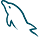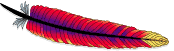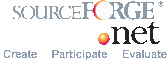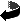Powered by© February 2007 Lorentz JÄNTSCHIUp

# Confidence intervals for a binomial proportion.

 Method: Wald WaldC AC (Agresti-Coull) ACC1 (Agresti-Coull) ACC2 (Agresti-Coull) Wilson WilsonC ArcS ArcSc1 ArcSc2 ArcSc3 Pau_Tak (approximate Clopper-Pearson) Logit LogitC BetaC00 BetaC10 BetaC01 (Clopper-Pearson - better) BetaE01 (Clopper-Pearson - original) BetaC11 (Bayes*) BetaCJ0 (Jeffreys) BetaCJ1 (Binomial Bayes-) BetaCJ2 (Binomial Jeffreys+) BetaCJA (Binomial 1*2) Optimized N_min: N_max: Display: Err(M,n,X) Ass(M,n) Avg(Ass(M,n))
Binomial Distributed Variable Confidence Intervals References (some of them):
 Name Method Reference Wald Wald Abraham WALD, Contributions to the Theory of Statistical Estimation and Testing Hypothesis, The Annals of Mathematical Statistics, p. 299-326, 1939. WaldC Wald cc (?) Brown D.L., Cai T.T., DasGupta A., Interval estimation for a binomial proportion, Statistical Science, 16, p. 101-133, 2001. AC Agresti-Coull Alan AGRESTI, Brent A. COULL, Approximate is better than 'exact' for interval estimation of binomial proportions, The American Statistician, 52, p. 119-126, 1998. ACC1 Agresti-Coull cc (?) Brown D.L., Cai T.T., DasGupta A., Interval estimation for a binomial proportion, Statistical Science, 16, p. 101-133, 2001. ACC2 Agresti-Coull cc [New correction] Wilson Wilson Edwin Bidwell WILSON, Probable Inference, the Law of Succession, and Statistical Inference, Journal of the American Statistical Association, 22, p. 209-212, 1927. WilsonC Wilson cc Robert G. NEWCOMBE, Two-Sided Confidence Intervals for the Single Proportion: Comparison of Seven Methods, Statistics in Medicine, 17, p. 857-872, 1998. ArcS ArcSine J. R. Anderson, L. Bernstein, M. C. Pike, Approximate Confidence Intervals for Probabilities of Survival and Quantiles in Life-Table Analysis, Biometrics, 38(2), p. 407-416, 1982. ArcSC1 ArcSine cc (?) Brown D.L., Cai T.T., DasGupta A., Interval estimation for a binomial proportion, Statistical Science, 16, p. 101-133, 2001. ArcSC2 ArcSine cc (?) Brown D.L., Cai T.T., DasGupta A., Interval estimation for a binomial proportion, Statistical Science, 16, p. 101-133, 2001. ArcSC3 ArcSine cc [New correction] Pau_Tak Paulson-Takeuchi Fujino Y., Approximate binomial confidence limits, Biometrika, 67(3), p. 677-681, 1980. Logit Logit (Woolf) Woolf B., On estimating the relation between blood group and disease, Annals of Human Genetics, 19, p. 251-253, 1955. LogitC Logit (Gart) Gart J. J., Alternative analyses of contingency tables, Journal of Royal Statistical Society, B28, p. 164-179, 1966. BetaC11 Bayes (Fisher) Fisher R. A., Statistical Methods for Scientific Inference, Oliver and Boyd, Edinburgh, 1956. BetaC01 Clopper-Pearson Clopper C., Pearson S., The use of confidence or fiducial limits illustrated in the case of the binomial, Biometrika, 26, p. 404-413, 1934. BetaCJ0 Jeffreys Jeffreys H., Theory of Probability (3rd Ed), Clarendon Press, Oxford, 1961. BetaC00 Beta cc [New correction] BetaC10 Beta cc [New correction] BetaCJ1 Beta cc [New correction] BetaCJ2 Beta cc [New correction] Optimized OptB [New correction] Sorana D. BOLBOACA, Lorentz JÄNTSCHI, Optimized Confidence Intervals for Binomial Distributed Samples, International Journal of Pure and Applied Mathematics, accepted (August 2, 2007), in press (Volume 40, Issue 3).